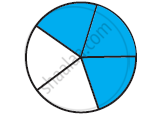Share

# Estimate What Part of the Figure is Coloured and Hence Find the per Cent Which is Coloured - 2 - CBSE Class 7 - Mathematics

ConceptConcept of Estimation

#### Question

Estimate what part of the figure is coloured and hence find the per cent which is coloured#### Solution

Here, 3 parts out of 5 equal parts are shaded which represents the fraction 3/53/5 = 3/5 xx 100 % = 60 %

Is there an error in this question or solution?

#### APPEARS IN

NCERT Solution for Mathematics for Class 7 (2018 to Current)
Chapter 8: Comparing Quantities
Ex. 8.20 | Q: 3.2 | Page no. 165
Solution Estimate What Part of the Figure is Coloured and Hence Find the per Cent Which is Coloured - 2 Concept: Concept of Estimation.
S